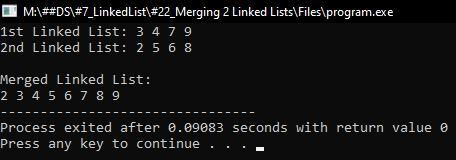# How to Merge two Linked Lists

## How to Merge two Linked Lists in C Language with Examples

In this article, I am going to discuss How to Merge two Linked Lists in C Language with Examples. Please read our previous article, where we discussed How to Concatenate two Linked Lists in C Language with Examples.

##### How to Merge two Linked Lists in C Language?

Now, let us discuss the merging of 2 linked lists. Let us first understand what is Merging.

##### Merging:

Merging is the process of combining two sorted lists into a single sorted list.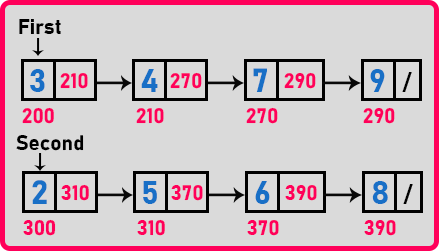Here we have two sorted linked lists and we will combine these two into a single sorted list. If you remember for merging two arrays, we require one more array but in linked lists, it’s not necessary. If you want you can take an extra linked list otherwise you can combine these two and make it a single linked list. You don’t need an extra linked list. We will explain the procedure of how merging is done over the linked list. So let us look at the procedure for Merging two Linked Lists in C Language.

##### Procedure for Merging two Linked Lists:

For merging we require one more pointer let us call it ‘third’. And to help this third pointer, we will take one more pointer that is ‘last’. So, we need two pointers. One is the ‘third’ that will be pointing to the first node of the combined linked list which is the merged linked list. And also, we will use one more point that is ‘last’. This will be pointing to the last node of that merged linked list.

The procedure is to compare the element of the first node in the first linked list and the element of the first node in the second linked list. Which one is smaller?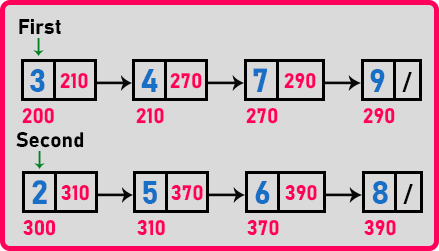The second linked list element is a smaller one that is ‘2’ is less than ‘3’. So, bring ‘last’ as well as ‘third’ pointer on the second list’s first node as,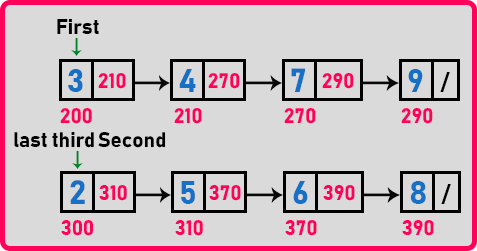Now move ‘second’ to the next node and make previous as null (last->next = NULL). We will write ‘second’ as ‘s’ and ‘first’ as ‘f’,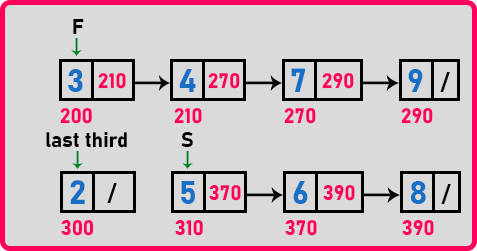Now we can see that the second linked list is having only 3 nodes. Then what about the previous node? The previous node is also there but it is the first node of the merged linked list where ‘third’ and ‘last’ are pointing.

Now again compare the node of the second linked list with the first list that is ‘3’ is smaller than ‘5’. So ‘last->next’ should point on the ‘3’ node and bring the last on the ‘3’ node. Then move ‘f’ to the next node and make ‘last->next’ as NULL,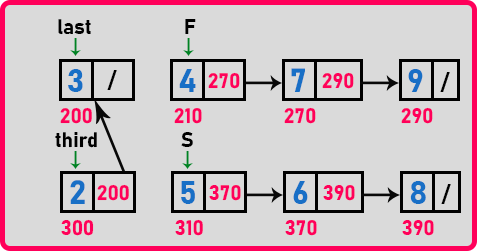Now merged linked has two nodes ‘2’ and ‘3’. Again compare ‘S’ and ‘F’, now ‘4’ is smaller than ‘5’ so make ‘last->next’ on ‘4’ and make last on ‘4’. Then move ‘F’ on the next node and make ‘last->next’ to NULL,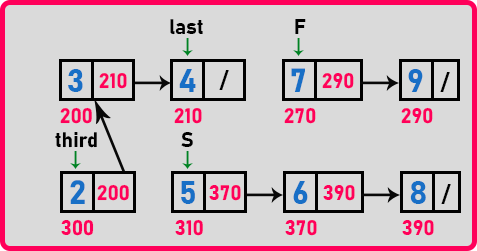Here the merged linked list has 3 nodes. Now ‘5’ is smaller than ‘7’ so make ‘last->next’ on ‘5’ and make last on ‘5’. Then move ‘S’ on the next node and make ‘last->next’ to NULL,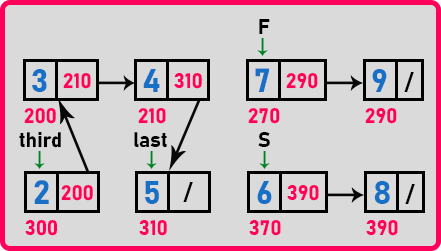Here the merged linked list has 4 nodes. Now ‘6’ is smaller than ‘7’ so make ‘last->next’ on ‘6’ and make last on ‘6’. Then move ‘S’ on the next node and make ‘last->next’ to NULL,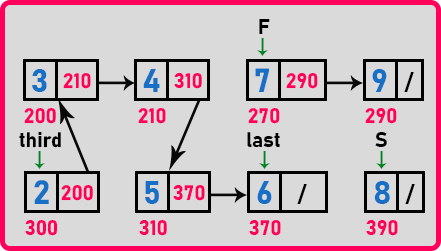By looking at the linked list, ‘6’ will point to ‘7’ and ‘7’ will point to ‘8’ as,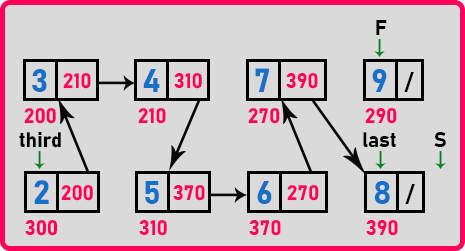Here ‘S’ is pointing to null, which means the second linked list has ended so we have to append all the nodes of another linked list if that has not ended. If any of the lists become null then we have to stop there and point the ‘last’ pointer on another list. In this way, we will merge the two linked lists. Final merged linked list: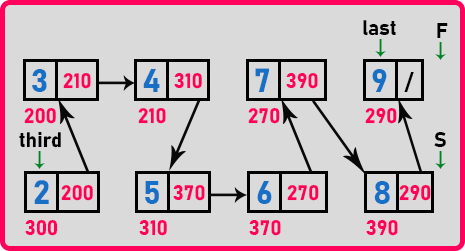So, this is the merged linked list. Now let us write pseudo code to perform merging in two linked lists.

##### Pseudo Code for Merging two Linked Lists:
```if(first->data < second->data){
third = last = first;
first = first->next;
last->next = NULL;
}
else{
third = last = second;
second = second->next;
last->next = NULL;
}
while(first != NULL && second != NULL){
if(first->data < second->data){
last->next = first;
last = first;
first = first->next;
last->next = NULL;
}
else{
last->next = second;
last = second;
second = second->next;
last->next = NULL;
}
}
if(first != NULL) last->next = first;
else last->next = second;
```

Now let us write the full program for merging two linked lists.

##### Program for Merging two Linked Lists using C Language:
```#include <stdio.h>
#include <stdlib.h>

struct Node
{
int data;
struct Node *next;
} *temp = NULL, *first = NULL, *second = NULL, *third = NULL, *last = NULL;

struct Node* Create (int A[], int n)
{
int i;
struct Node *t, *last;
temp = (struct Node *) malloc(sizeof(struct Node));
temp->data = A;
temp->next = NULL;
last = temp;

for (i = 1; i < n; i++)
{
t = (struct Node *) malloc(sizeof(struct Node));
t->data = A[i];
t->next = NULL;
last->next = t;
last = t;
}
return temp;
}

void Display(struct Node *p)
{
while (p != NULL)
{
printf ("%d ", p->data);
p = p->next;
}
}

void Merge(struct Node *first, struct Node *second)
{
if (first->data < second->data)
{
third = last = first;
first = first->next;
last->next = NULL;
}
else
{
third = last = second;
second = second->next;
last->next = NULL;
}

while (first != NULL && second != NULL)
{
if (first->data < second->data)
{
last->next = first;
last = first;
first = first->next;
last->next = NULL;
}
else
{
last->next = second;
last = second;
second = second->next;
last->next = NULL;
}
}

if (first != NULL)
last->next = first;
else
last->next = second;
}

int main()
{
int A[] = { 3, 4, 7, 9 };
int B[] = { 2, 5, 6, 8 };
first = Create (A, 4);
second = Create (B, 4);

Display (first);

Display (second);

Merge (first, second);